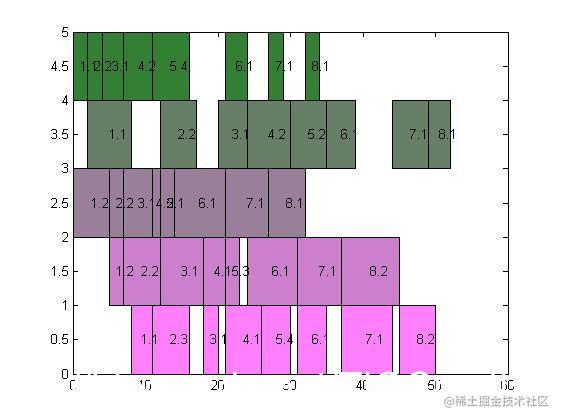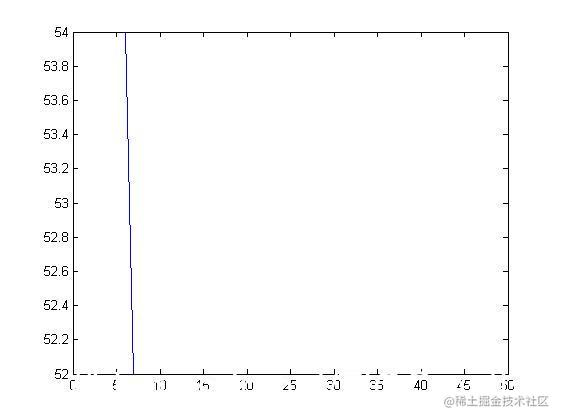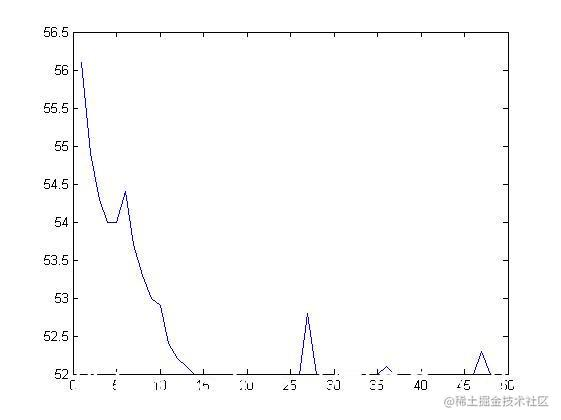# 【车间调度】基于遗传算法求解混合流水车间调度最优问题matlab源码

## 一、简介

1 遗传算法概述

2 遗传算法的特点和应用

（1）以决策变量的编码作为运算对象。传统的优化算法往往直接利用决策变量的实际值本身来进行优化计算，但遗传算法是使用决策变量的某种形式的编码作为运算对象。这种对决策变量的编码处理方式，使得我们在优化计算中可借鉴生物学中染色体和基因等概念，可以模仿自然界中生物的遗传和进化激励，也可以很方便地应用遗传操作算子。
（2）直接以适应度作为搜索信息。传统的优化算法不仅需要利用目标函数值，而且搜索过程往往受目标函数的连续性约束，有可能还需要满足“目标函数的导数必须存在”的要求以确定搜索方向。遗传算法仅使用由目标函数值变换来的适应度函数值就可确定进一步的搜索范围，无需目标函数的导数值等其他辅助信息。直接利用目标函数值或个体适应度值也可以将搜索范围集中到适应度较高部分的搜索空间中，从而提高搜索效率。
（3）使用多个点的搜索信息，具有隐含并行性。传统的优化算法往往是从解空间的一个初始点开始最优解的迭代搜索过程。单个点所提供的搜索信息不多，所以搜索效率不高，还有可能陷入局部最优解而停滞；遗传算法从由很多个体组成的初始种群开始最优解的搜索过程，而不是从单个个体开始搜索。对初始群体进行的、选择、交叉、变异等运算，产生出新一代群体，其中包括了许多群体信息。这些信息可以避免搜索一些不必要的点，从而避免陷入局部最优，逐步逼近全局最优解。
（4） 使用概率搜索而非确定性规则。传统的优化算法往往使用确定性的搜索方法，一个搜索点到另一个搜索点的转移有确定的转移方向和转移关系，这种确定性可能使得搜索达不到最优店，限制了算法的应用范围。遗传算法是一种自适应搜索技术，其选择、交叉、变异等运算都是以一种概率方式进行的，增加了搜索过程的灵活性，而且能以较大概率收敛于最优解，具有较好的全局优化求解能力。但，交叉概率、变异概率等参数也会影响算法的搜索结果和搜索效率，所以如何选择遗传算法的参数在其应用中是一个比较重要的问题。

、机器人学、图像处理（图像恢复、图像边缘特征提取…)、人工生命、遗传编程、机器学习。

3 遗传算法的基本流程及实现技术

3.1 遗传算法的基本流程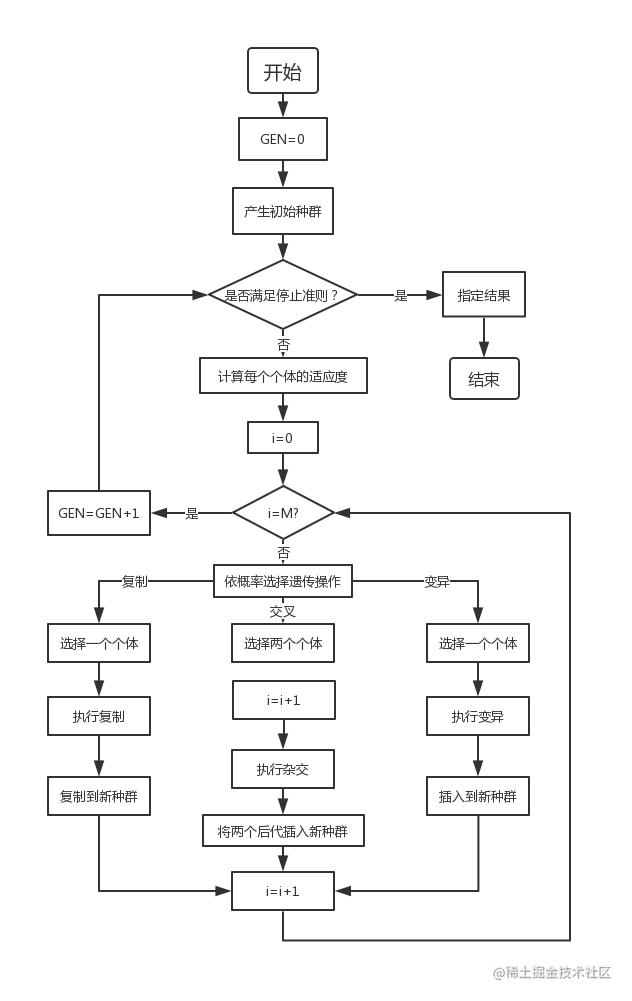3.2 遗传算法的实现技术

3.2.1 编码
（1）二进制编码

（2）其他编码方法

3.2.2 适应度函数

3.2.3选择算子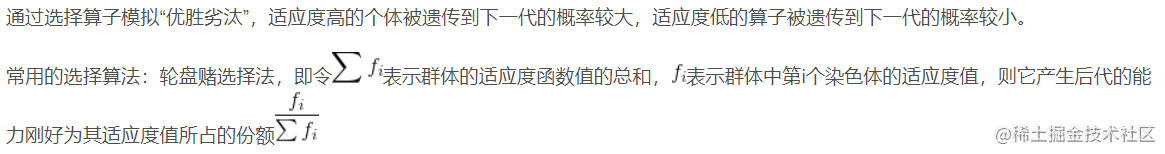3.2.4 交叉算子

3.2.5 变异算子

3.2.6 运行参数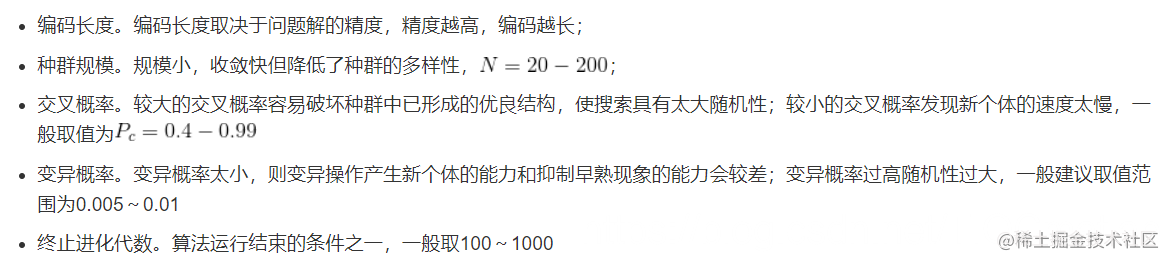4 遗传算法的基本原理
4.1 模式定理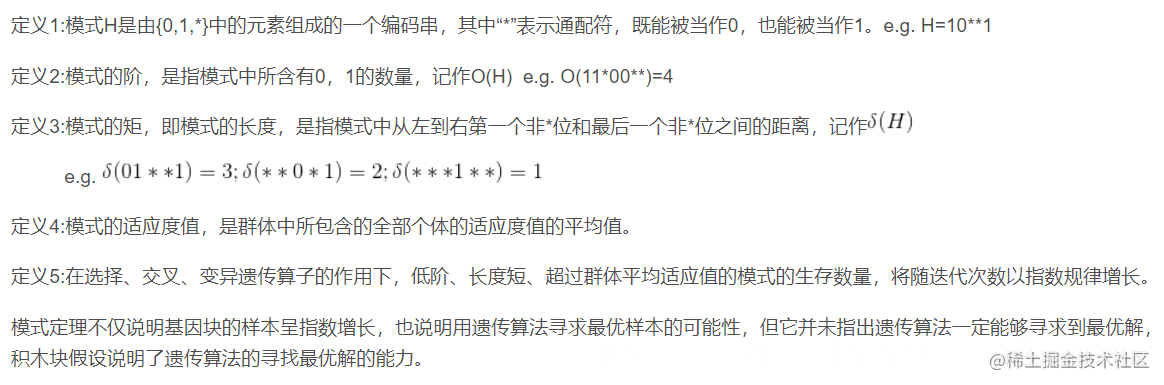4.2 积木块假设

## 二、源代码

``````unction [Zp,Y1p,Y2p,Y3p,Xp,LC1,LC2]=JSPGA(M,N,Pm,T,P)

%----------------------------------------------------------------
%   JSPGA.m 流水车间调度遗传算法
%----------------------------------------------------------------
% 输入参数列表
%    M  遗传进化迭代次数
%    N  种群规模（偶数）
%    Pm 变异概率
%    T  m*n的矩阵，存储m个工件n个工序的加工时间
%    P  1*n的向量，n个工序中，每一个工序所具有的机床数目
%----------------------------------------------------------------
% 输出参数列表
%   Zp   最优的Makespan值
%   Y1p  最优方案中，各工件各工序的开始时刻，可用来绘制甘特图
%   Y2p  最优方案中，各工件各工序的结束时刻
%   Y3p  最优方案中，各工件各工序使用的机器编号
%   Xp   最优决策变量的值，决策变量是一个实数编码的m*n矩阵
%   LC1  收敛曲线1，各代最优个体适应值的记录
%   LC2  收敛曲线2，各代群体平均适应值的记录
% 最后程序将绘制三幅图片：两条收敛曲线和各工件调度的甘特图

%----------------------------------------------------------------
% 第一步：变量初始化
[m,n]=size(T);  %m是总工件数，n是总工序数
Xp=zeros(m,n);  %最优决策变量
LC1=zeros(1,M); %收敛曲线1
LC2=zeros(1,N); %收敛曲线2
%----------------------------------------------------------------
% 第二步：随机产生初始种群farm
farm=cell(1,N); %采用细胞结构存储种群
for k=1:N
X=zeros(m,n);
for j=1:n
for i=1:m
X(i,j)=1+(P(j)-eps)*rand; %eps默认eps(1)表示matlab做能表达的1的精度即1+0.9*eps=1
end
end
farm{k}=X;
end
counter=0;  %设置迭代计数器
while counter<M     %停止条件为打到最大迭代次数
%----------------------------------------
% 第三步：交叉
newfarm=cell(1,N);  %交叉产生的新种群存在其中
Ser=randperm(N);    %randperm(N)返回1：N的随机排序向量
for i=1:2:(N-1)
A=farm{Ser(i)};     %父代个体
B=farm{Ser(i+1)};   %父代相邻个体
Manner=unidrnd(2);  %随机选择交叉方式（横向交叉/纵向交叉）  unidrnd(N)返回1：N的离散随机数
if Manner==1
cp=unidrnd(m-1);    %随机选择交叉点
%双亲双子单点交叉
a=[A(1:cp,:);B((cp+1):m,:)];    %子代个体
b=[B(1:cp,:);A((cp+1):m,:)];
else
cp=unidrnd(n-1);    %随机选择交叉点
a=[A(:,1:cp),B(:,(cp+1):n)];    %双亲双子单点交叉
b=[B(:,1:cp),A(:,(cp+1):n)];
end
newfarm{i}=a;   %交叉后的子代存入newfarm
newfarm{i+1}=b;
end
%新旧种群合并
FARM=[farm,newfarm];
%----------------------------------------
% 第四步：选择复制
FITNESS=zeros(1,2*N);
fitness=zeros(1,N);
plotif=0;
for i=1:(2*N)
X=FARM{i};
Z=COST(X,T,P,plotif);   %调用计算费用的子函数，计算适应值
FITNESS(i)=Z;
end
%选择复制采取两两随机配对竞争的方式，具有保留最优个体的能力
Ser=randperm(2*N);
for i=1:N
f1=FITNESS(Ser(2*i-1));
f2=FITNESS(Ser(2*i));
if f1<=f2
farm{i}=FARM{Ser(2*i-1)};
fitness(i)=FITNESS(Ser(2*i-1));
else
farm{i}=FARM{Ser(2*i)};
fitness(i)=FITNESS(Ser(2*i));
end
end
%记录最佳个体和收敛曲线
minfitness=min(fitness);    %找出10个种群中最小的个体
meanfitness=mean(fitness);  %10个种群的平均适应值
LC1(counter+1)=minfitness;  %收敛曲线1，各代最优个体适应值的记录
LC2(counter+1)=meanfitness; %收敛曲线2，各代群体平均适应值的记录
pos=find(fitness==minfitness); %记录10个种群中最小个体的位置
Xp=farm{pos(1)};            %将最小个体位置的第一个排序结果记录
%----------------------------------------
% 第五步：变异
for i=1:N
if Pm>rand %变异概率为Pm

X(I,J)=1+(P(J)-eps)*rand;
farm{i}=X;
end
end
farm{pos(1)}=Xp;        %变异之后，具有保留最优个体的能力
counter=counter+1;
end
% 第一步：变量初始化
[m,n]=size(X);
Y1p=zeros(m,n);
Y2p=zeros(m,n);
Y3p=zeros(m,n);
%----------------------------------------------------------------
% 第二步：计算第一道工序的安排
Q1=zeros(m,1);
Q2=zeros(m,1);
R=X(:,1);   %取出第一道工序
Q3=floor(R);    %向下取整即得到各工件在第一道工序使用的机器编号
% 下面计算各工件第一道工序的开始时刻和结束时刻
for i=1:P(1)        %取出机器编号
pos=find(Q3==i);%取出使用编号为i的机器为其加工的工件编号 find返回向量/矩阵中的位置
lenpos=length(pos);
if lenpos>=1
Q1(pos(1))=0;
Q2(pos(1))=T(pos(1),1);
if lenpos>=2
for j=2:lenpos
Q1(pos(j))=Q2(pos(j-1));
Q2(pos(j))=Q2(pos(j-1))+T(pos(j),1);
end
end
end
end
Y1p(:,1)=Q1;
Y2p(:,1)=Q2;
Y3p(:,1)=Q3;
%----------------------------------------------------------------
% 第三步：计算剩余工序的安排
for k=2:n
R=X(:,k);       %取出第k道工序
Q3=floor(R);    %向下取整即得到各工件在第k道工序使用的机器编号
%下面计算各工件第k道工序的开始时刻和结束时刻
for i=1:P(k)    %取出机器编号
pos=find(Q3==i);    %取出使用编号为i的机器为其加工的工件编号
lenpos=length(pos);
if lenpos>=1
EndTime=Y2p(pos,k-1);   %取出这些机器在上一个工序中的结束时刻
POS=zeros(1,lenpos);    %上一个工序完成时间由早到晚的排序
for jj=1:lenpos
MinEndTime=min(EndTime);
ppp=find(EndTime==MinEndTime);
POS(jj)=ppp(1);
EndTime(ppp(1))=Inf;
end
%根据上一个工序完成时刻的早晚，计算各工件第k道工序的开始时刻和结束时刻
Q1(pos(POS(1)))=Y2p(pos(POS(1)),k-1);
Q2(pos(POS(1)))=Q1(pos(POS(1)))+T(pos(POS(1)),k);%前一个工件的结束时刻
if lenpos>=2
for j=2:lenpos
Q1(pos(POS(j)))=Y2p(pos(POS(j)),k-1);    %预定的开始时刻为上一个工序的结束时刻
Q2(pos(POS(j)))=Q1(pos(POS(j)))+T(pos(POS(j)),k);%前一个工件的结束时刻
if Q1(pos(POS(j)))<Q2(pos(POS(j-1)))   %如果比前面的工序的结束时刻还早
Q1(pos(POS(j)))=Q2(pos(POS(j-1)));
Q2(pos(POS(j)))=Q1(pos(POS(j)))+T(pos(POS(j)),k);%前一个工件的结束时刻
end
end
end
end
end

## 三、运行结果